# Cuboctahedron atop Truncated Cube

Cuboctahedron atop Truncated Cube (cuboctahedron || cube), or K4.129 among Dr. Richard Klitzing's segmentochora, is a 4D CRF polytope that consists of a cuboctahedron and a truncated cube in parallel hyperplanes, connected to each other by 8 triangular prisms and 6 square cupolae. It has a total of 16 cells, 64 polygons (28 triangles, 30 squares, 6 octagons), 84 edges, and 36 vertices.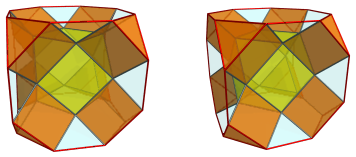Besides the defining construction of taking the convex hull of a cuboctahedron and a truncated cube placed in parallel hyperplanes, K4.129 can also be constructed as a Stott expansion of the cubical pyramid (a cantellation).

Eight copies of K4.129 can be attached to the truncated tesseract to form the octa-augmented truncated tesseract, in which adjacent square cupolae lie in coincident hyperplanes and thus merge into square orthobicupolae (J28).

## Structure

The structure of K4.129 is quite simple. We shall explore it using its parallel projections into 3D:The above image shows the cuboctahedral cell. Its triangular faces are joined to 8 prisms, shown next: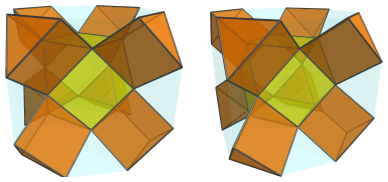The square faces of the cuboctahedron are joined to 6 square cupolae: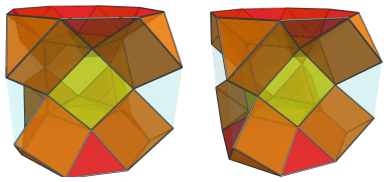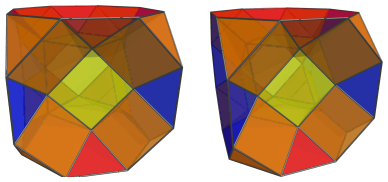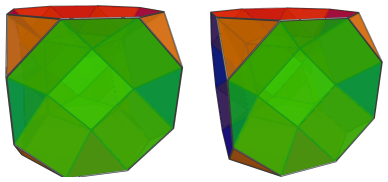Finally, closing up the entire shape is the truncated cube: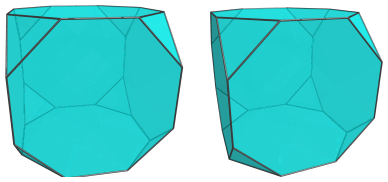For clarity, we show only the truncated cube and omit the cells previously seen.

## Coordinates

The Cartesian coordinates of K4.129 with edge length 2 are:

• (0, ±√2, ±√2, 1)
• (±√2, 0, ±√2, 1)
• (±√2, ±√2, 0, 1)
• (±1, ±(1+√2), ±(1+√2), 0)
• (±(1+√2), ±1, ±(1+√2), 0)
• (±(1+√2), ±(1+√2), ±1, 0)

Last updated 18 Jun 2019.